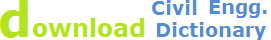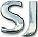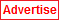Watch Differential equations and Numerical Analysis Videos , Advanced Engineering Mathematics kreysig

Home GSM Differential Equations Solutions

 Differential Equations Advanced Engineering Mathematics Course OutlinesSolution of differential equations course and hints for solving equations on differential equations and partial differential equations, elementary, non linear, projectile and homogenous Diff. Eqs. . A full course and help for the learners. This is a simple tutor to differential equations and how to solve differential equations.

This course includes a low quality as well as high quality solutions. You can download any of them as depending on the speed of you internet connection, as the high quality solutions are a bit larger in a size.
The Solutions are for the exercises in the book named Advanced Engineering Mathematics. 8th Edition, By Erwin Kreysig.About Us | Site Map | Privacy Policy | Contact Us |© 2014 WebTechTix